Knowledge

# Factor Tree

Know it All about Factor Tree

We make a list of all the people who came before us when we make a family tree. They are commonly known as family trees because when each ancestor gives way to more ancestors, they begin to branch out. On the other hand, a factor tree depicts the numbers that combine to form a larger number, and it branches out as it grows since each resulting factor gives way to its own set of factors. A factor tree, in other words, is a method for breaking down any number into its prime factors.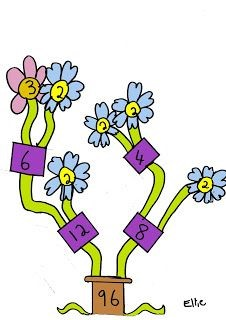In this guide, you will learn what a factor tree is. Moreover, you will also get to know why it's beneficial along with a few easy tips to make one.

### Part 1: What is a Factor Tree?

Before going into details about what a factor tree is, you must first know what a factor is, when it comes to math. Factors are simply numbers that can be multiplied together to produce a specific result. When we look at 2 * 3 = 6, for instance, 2 and 3 are factors of 6. However, we might assume that 1 * 6 = 6. So there are four factors of number 6 are 1, 2, 3, and 6. With large numbers, this factoring business could get complicated because, in general, the bigger a number is, the more factors it will have. That's where the factor tree comes into the picture.

In a factor tree, we find the factors of a number. Then, we see the factors of those resulting numbers until we finally can't factor anymore. All the numbers at the end are the prime factors of the original number.

For instance, here is a factor tree of the number 48. It shows that 48 = 2 x 2 x 2 x 2 x 3.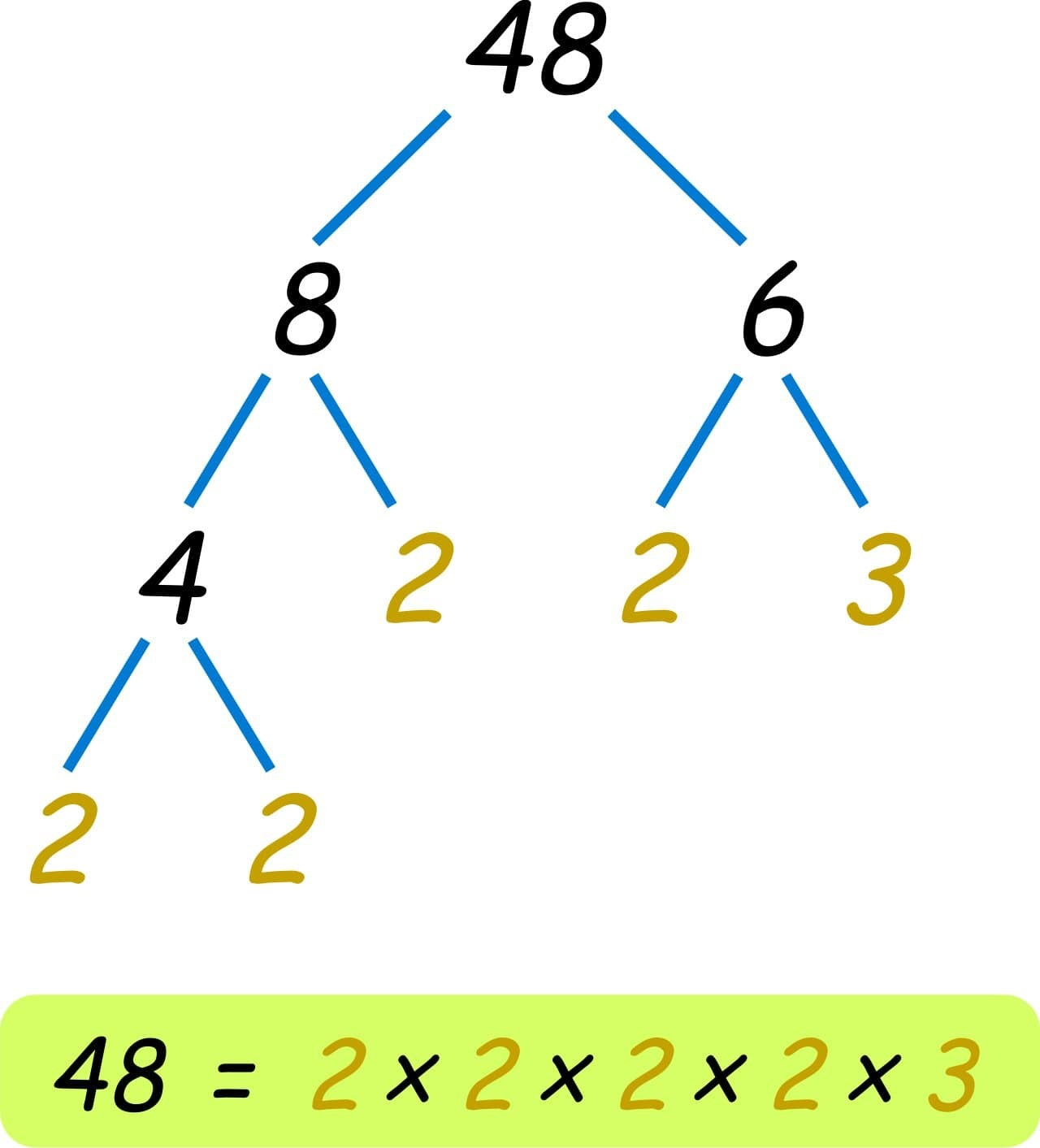### Part 2: Purpose and Benefits of a Factor Tree

A factor tree is a convenient way to list down all the prime factors of a number that is greater than one. Factoring, or listing all of an integer's prime factors, is a valuable skill that also aids in the solution of math problems. Factoring with a factor tree has quite many benefits.

For example, listing all of the two integers' prime factors is one way to find their GCD (Greatest Common Divisor) or LCM (Least Common Multiple). The GCD is then the sum of all common factors, while the LCM is the sum of all remaining factors.

The factor tree also represents the order in which the factors were discovered. Each number can have more than one factor. Moreover, for any number, different factor trees generate the exact prime factorization. An example of it is as below. You can see the three different factor trees for the number 108 producing the same results.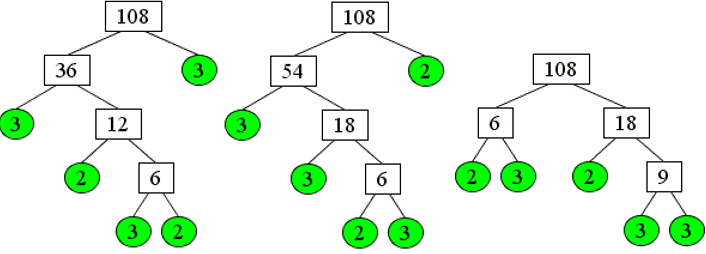### Part 3: When to Use a Factor Tree?

As explained above, you can use a factor tree to determine all possible prime factors of a number. Similarly, you can also follow an easy method to find the greatest common factor (GCF) and least common multiple (LCM) of two or more than two numbers using a factor tree.

• To find the GCF of two numbers, draw a factor tree for both of the numbers. Look for the common numbers among the resulting prime factors of both the trees. The GCF will be the common numbers multiplied together. For example: if 2 and 3 are common factors of two numbers, the GCF will be 2 x 3 or 6.
• To find the LCM, begin the same way by drawing a factor tree for both numbers. Again, look at the resulting prime factors. The LCM will be the product of the largest number of each prime factor appearing on each list. For example: if the largest multiples are as follows, the LCM will be 360.
• 2 x 2 x 2 x 3 x 3 x 5 = 360

### Part 4: How to Create a Factor Tree

Creating a factor tree comes with several benefits. You can either draw it manually or choose software for a better, quicker and more convenient experience. Make sure to use a reliable platform like EdrawMax. Here you can create powerful illustrations and graphic organizers in the form of creative charts, diagrams, maps, and many more. To create a factor tree, follow the step-by-step tutorial below:

2.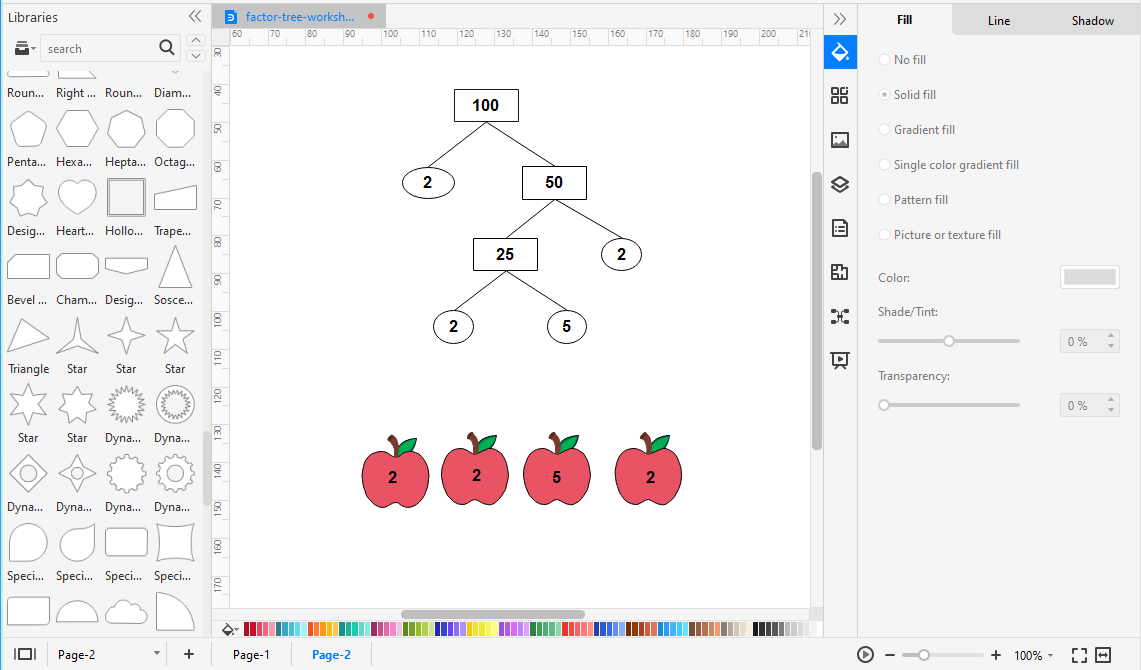3. Once done, open and choose from a wide range of already-drawn templates. Go to the search bar and type in for the visual diagrams to appear. Choose from the existing diagrams or click on the “+” to create your own from scratch.
4. This will take you to the drawing page. Once on it, use the Icon Library on the left side of the screen to use icons, drawings, and other visual tools. By clicking on the icon in the Symbol Library, you can check for more.
5.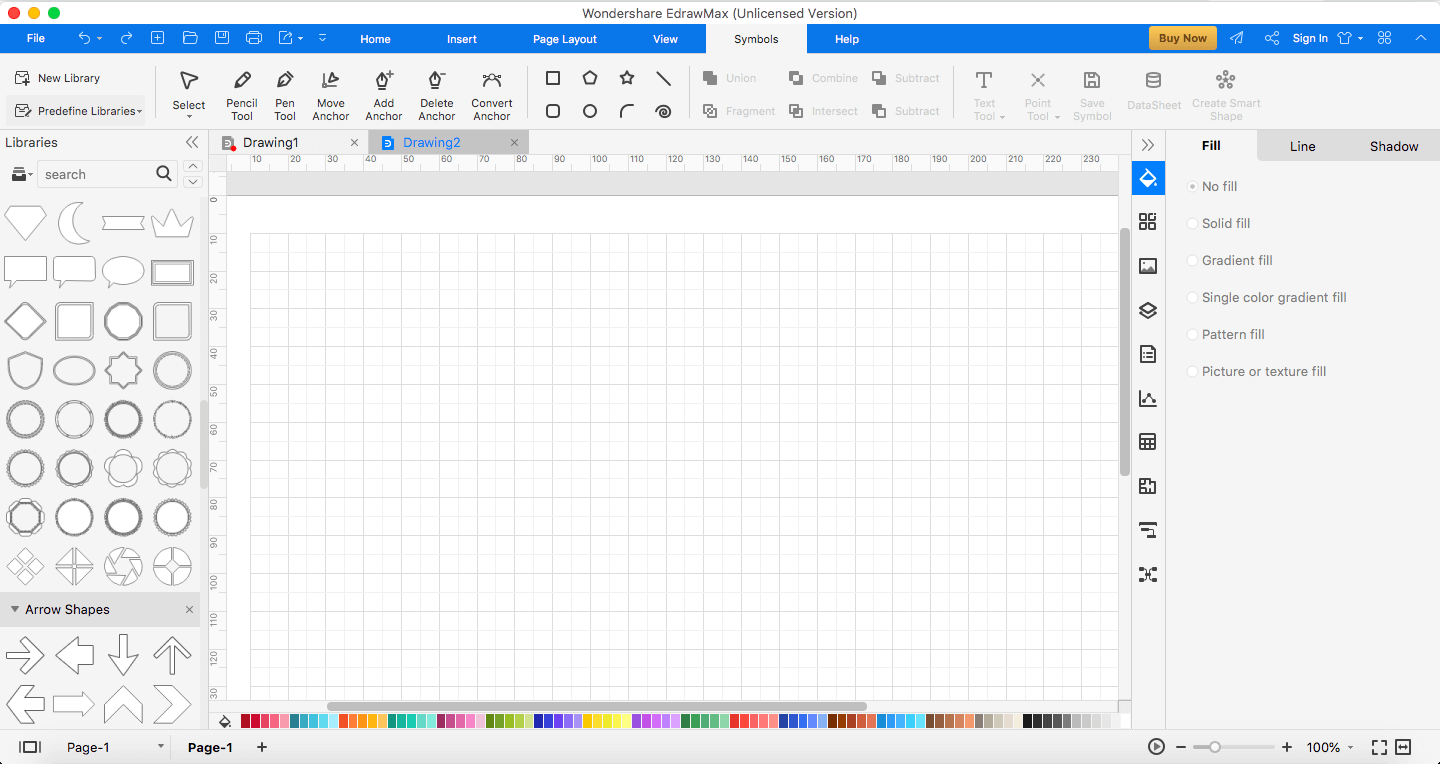6. Next, draw symbols on the drawing page and link them with connection lines. To add an object to your drawing list, select it and move it to the desired location on the page.
7.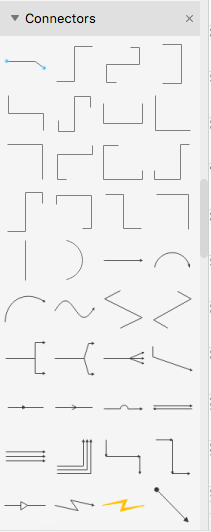8. Keep editing your diagram to perfection or until you are satisfied with the results.
9. Finally, close the format tab and make sure to save your file. You can either save it as a picture, a PDF, or a document.
10.## EdrawMax

##### All-in-One Diagram Software
Create more than 280 types of diagrams effortlessly
Start diagramming with various templates and symbols easily
• Superior file compatibility: Import and export drawings to various file formats, such as Visio
• Cross-platform supported (Windows, Mac, Linux, Web)Security Verified | Switch to Mac >>Security Verified | Switch to Windows >>

### Part 5: Tips for making a Factor Tree

Below are some tips for making a factor tree.

1. Use clear connection lines for a better visual representation
2. Any number can be split into prime factors in exactly one way, so the prime factorization would be the same regardless of how the number is factorized

### Part 6: Examples

Here is an example of the factor tree for the number 144. As you can see, the diagram contains prime factors of each of the resulting numbers. Furthermore, the yellow circles indicate that the numbers cannot be factorized anymore.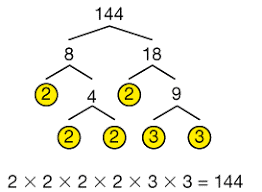As explained earlier, each number can be factored in a lot of ways. Therefore, here is an example of the factor tree for the number 30 in every possible way. Note that the resulting prime factors always have one 2, one 3, and one 5. So, it can be written in prime factorization as 2 x 3 x 5 and multiplied to get the result of 30.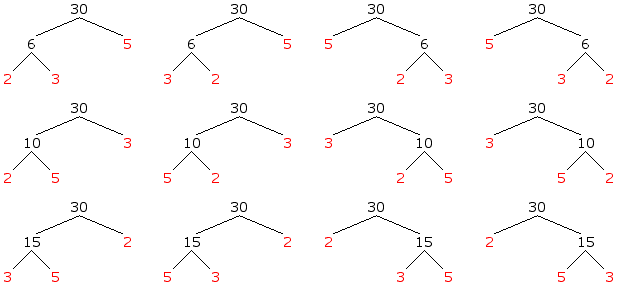A cartoon factor tree worksheet is shown below. The factor tree is located inside a green tree with a trunk under which students can fill in prime factors. This type of factor tree worksheet is ideal for primary students who are cartoon fans.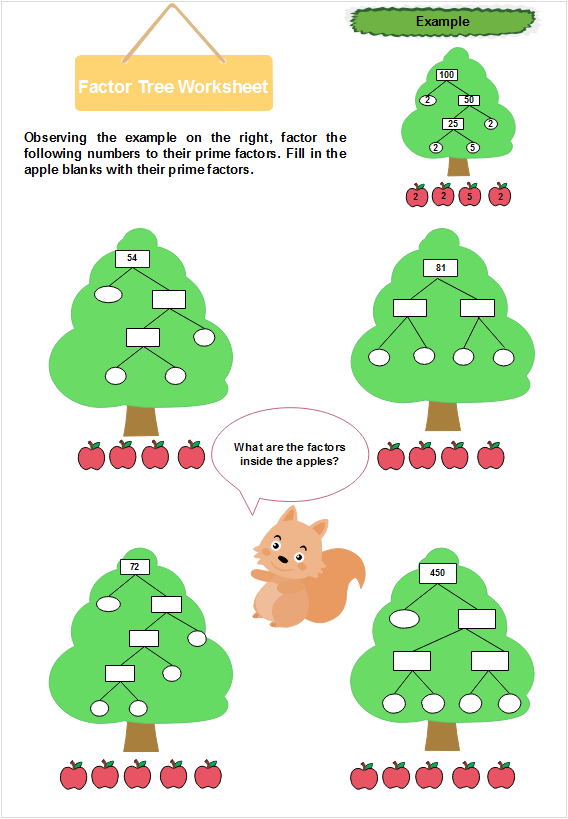### Part 7: Conclusion

Lastly, making a factor tree is an easy way to find all of a number's prime number factors. It becomes easier to perform more advanced tasks, such as discovering the greatest common factor or the least common multiple, once you've mastered factor trees.

With EdrawMax's amazing and helpful features, you can easily create a factor tree for you or your students' ease. Make sure to check out their website today!

Related Articles
Create a Factor Tree with EdrawMax. Try it Free!